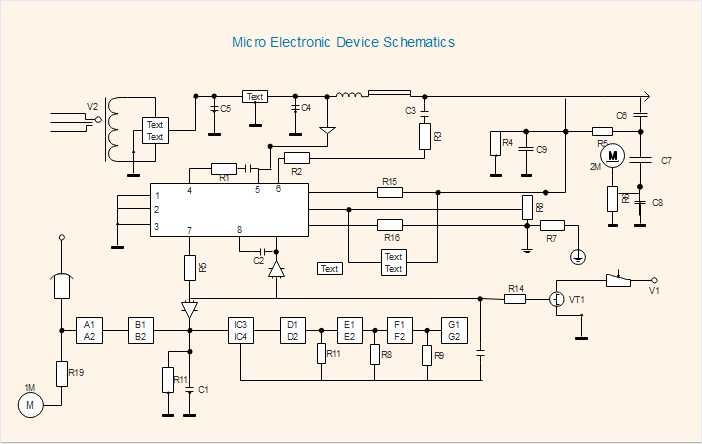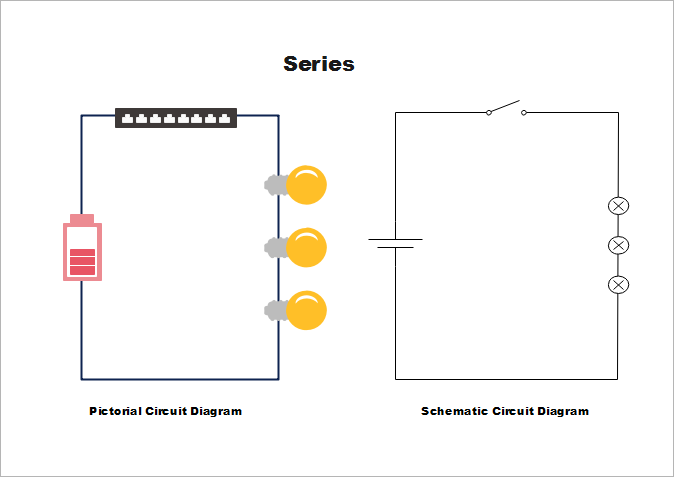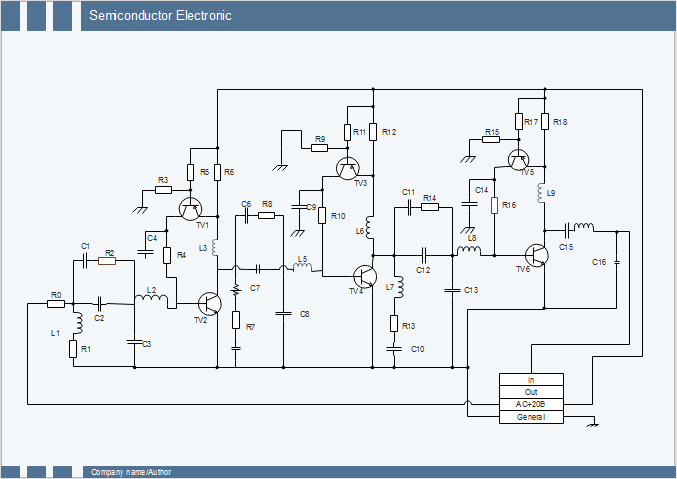Home
• Products
• Pricing
• Office Efficiency
• Project Management
• Graphic Design
• Engineering
• Education
• Special Industry
• Others

# Difference between Schematics and Circuit Diagrams

Schematics, circuit diagrams, wiring diagrams, electrical diagrams are commonly used engineering diagrams. You may have heard them very often, but they vary each other slightly. Refer to this page to learn the differences between schematics and circuit diagrams.

This article illustrates the differences between schematics and circuit diagrams, and may benefit you a lot in identifying the components of a system, tracing a circuit and even fixing electrical equipment. I'm sure that you are able to tell how schematics differ from circuit diagrams and the relationship between them.

### Schematics

A schematic, or schematic diagram, represents the elements of a system with abstract, graphic symbols instead of realistic pictures. A schematic focuses more on comprehending and spreading information rather than doing physical operations. For this reason, a schematic usually omits all details that are not relevant to the information it intends to convey, and may add unrealistic elements to help understand the features and relationships.

Electronic schematic to electronics is what recipe is to a chef. They tell you what ingredients to use and how to get the ingredients arranged and connected. Instead of explaining the recipe with detailed texts, a drawing is used to depict the construction of electronics. Electronic schematics consist of digital electronic symbols that represent each of the components used. On the following microelectronic device schematic diagram, the symbols are connected with lines that show you how to connect the components.### Circuit Diagram

A circuit diagram (also named electrical diagram, elementary diagram, electronic schematic) is a graphical representation of an electrical circuit. Circuit diagrams are widely used for the circuit design, construction, and maintenance of electrical and electronic equipment. Circuit diagrams can be divided into two categories - pictorial circuit diagram and schematic circuit diagram.

#### Pictorial Circuit Diagram

Pictorial diagrams are much easier to understand than schematic circuit diagrams. Connecting realistic electrical components with the wiring, a pictorial diagram makes it easy for viewers to identify the electrical components of a system immediately without professional knowledge required.#### Schematic Circuit Diagram

A schematic circuit diagram represents the electrical system in the form of a diagram that shows the main features or relationships but not the details. In a schematic circuit diagram, the presentation of electrical components and wiring does not completely correspond to the physical arrangements in the real device. If you want to understand a schematic diagram, you are required to master basic knowledge of electricity and physics as well as internationally standardized symbols. Look at the parallel circuits below, you may find that the battery is represented as two short lines, the lights are represented as a circle with a cross inside, and the wiring is represented as a line. This kind of circuit diagram is mainly used by electrical engineers. The following is a schematic circuit diagram of a semiconductor electronic.Schematics and circuit diagrams are both important engineering diagrams. Edraw all-in-one diagramming software is an excellent schematics and circuit diagrams maker. Free download the software to create your works.

#### Related Articles:

Circuit Diagram Software for Mac

Circuit Diagram Visio Alternative

Create Circuit Diagram for PPT